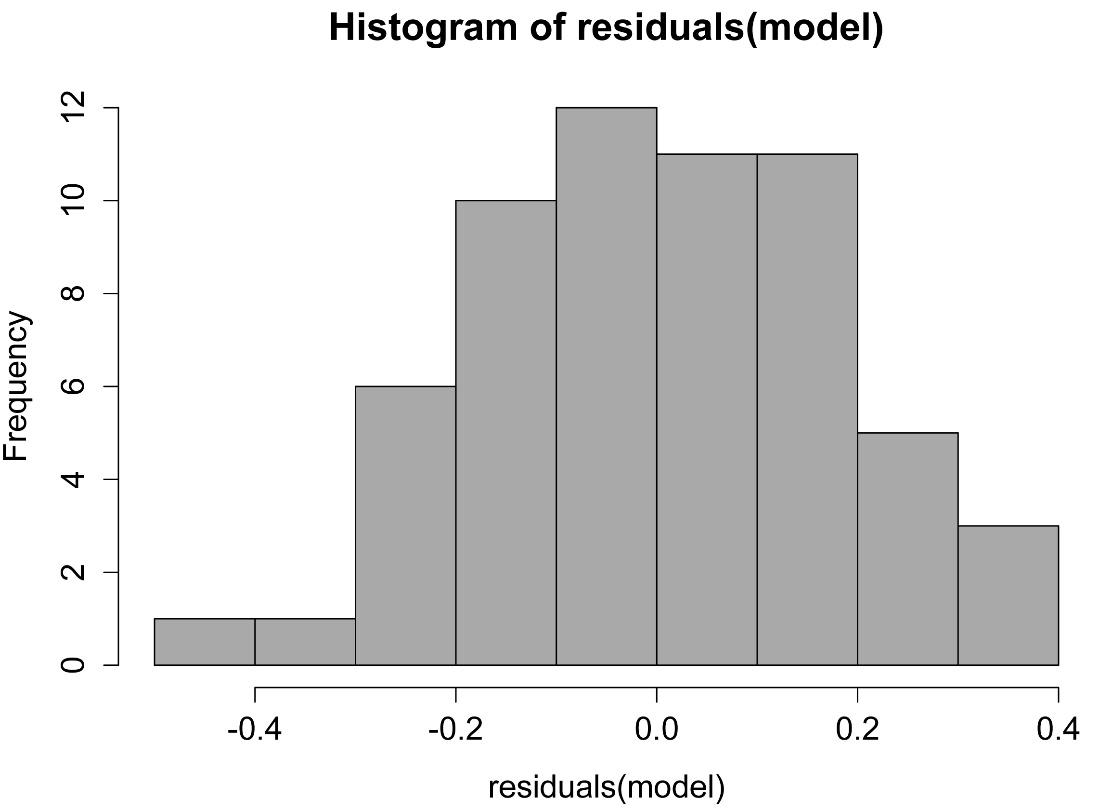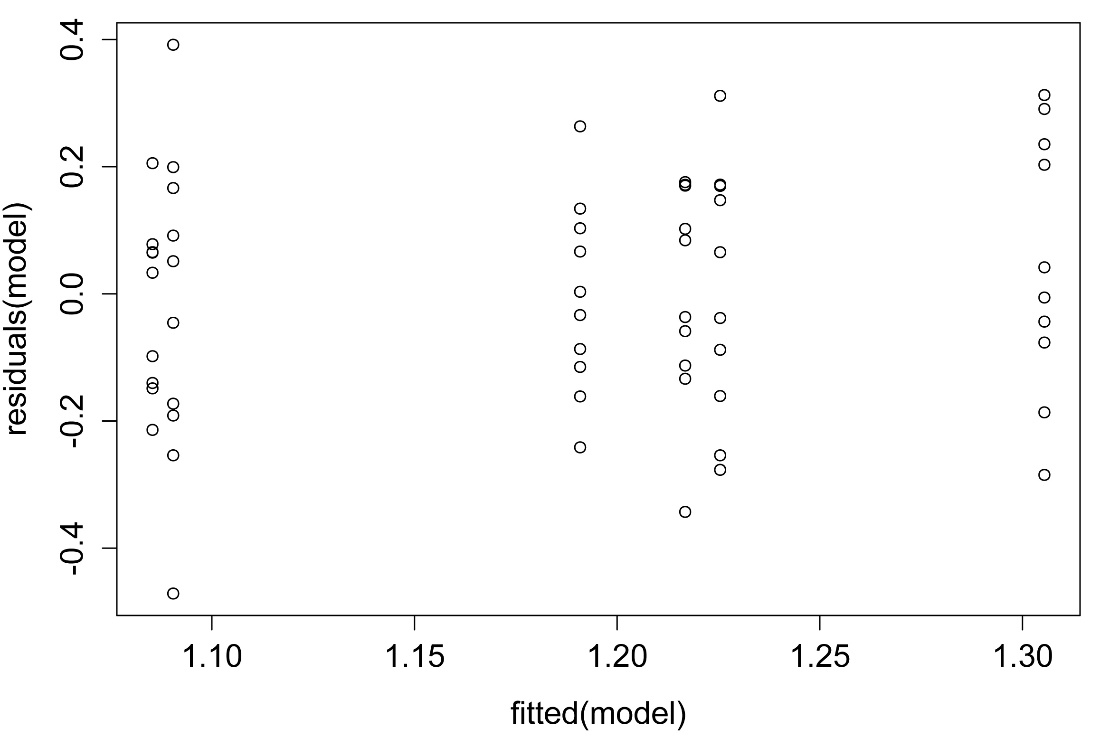## An R Companion for the Handbook of Biological Statistics

Salvatore S. Mangiafico

# Nested Anova

### Packages used in this chapter

The following commands will install these packages if they are not already installed:

if(!require(nlme)){install.packages("nlme")}
if(!require(multcomp)){install.packages("multcomp")}
if(!require(multcompView)){install.packages("multcompView")}
if(!require(lsmeans)){install.packages("lsmeans")}
if(!require(lme4)){install.packages("lme4")}
if(!require(lmerTest)){install.packages("lmerTest")}
if(!require(TukeyC)){install.packages("TukeyC")}

When to use it

Null hypotheses

How the test works

Partitioning variance and optimal allocation of resources

Unequal sample sizes

Assumptions

Example

Graphing the results

Similar tests

See the Handbook for information on these topics.

### How to do the test

#### Nested anova example with mixed effects model (nlme)

One approach to fit a nested anova is to use a mixed effects model.  Here Tech is being treated as a fixed effect, while Rat is treated as a random effect.  Note that the F-value and p-value for the test on Tech agree with the values in the Handbook.  The effect of Rat will be tested by comparing this model to a model without the Rat term.  The model is fit using the lme function in nlme.

### --------------------------------------------------------------
### Nested anova, SAS example, pp. 171-173
### --------------------------------------------------------------

Input =("
Tech  Rat Protein
Janet 1   1.119
Janet 1   1.2996
Janet 1   1.5407
Janet 1   1.5084
Janet 1   1.6181
Janet 1   1.5962
Janet 1   1.2617
Janet 1   1.2288
Janet 1   1.3471
Janet 1   1.0206
Janet 2   1.045
Janet 2   1.1418
Janet 2   1.2569
Janet 2   0.6191
Janet 2   1.4823
Janet 2   0.8991
Janet 2   0.8365
Janet 2   1.2898
Janet 2   1.1821
Janet 2   0.9177
Janet 3   0.9873
Janet 3   0.9873
Janet 3   0.8714
Janet 3   0.9452
Janet 3   1.1186
Janet 3   1.2909
Janet 3   1.1502
Janet 3   1.1635
Janet 3   1.151
Janet 3   0.9367
")

### Since Rat is read in as an integer variable, convert it to factor

Data\$Rat = as.factor(Data\$Rat)

library(nlme)

model = lme(Protein ~ Tech, random=~1|Rat,
data=Data,
method="REML")

anova.lme(model,
type="sequential",

numDF denDF  F-value p-value
(Intercept)     1    54 587.8664  <.0001
Tech            1     4   0.2677  0.6322

##### Post-hoc comparison of means

Note that “Tukey” here instructs the glht function to compare all means, not to perform a Tukey adjustment of multiple comparisons.

library(multcomp)

posthoc = glht(model,
linfct = mcp(Tech="Tukey"))

mcs = summary(posthoc,

mcs

### Adjustment options: "none", "single-step", "Shaffer",
###                     "Westfall", "free", "holm", "hochberg",
###                     "hommel", "bonferroni", "BH", "BY", "fdr"

Linear Hypotheses:
Estimate Std. Error z value Pr(>|z|)
Janet - Brad == 0 -0.05060    0.09781  -0.517    0.605

cld(mcs,
level=0.05,
decreasing=TRUE)

"a"   "a"

### Means sharing a letter are not significantly different

##### Post-hoc comparison of least-square means

Least squares means are adjusted for other terms in the model.  If the experimental design is unbalanced or there is missing data, the least square means may differ significantly from arithmetic means for treatments, but are generally more representative of the population means than the arithmetic means would be.

Note that the adjustments for multiple comparisons (adjust =”tukey”) appears in both the lsmeans and cld functions.

library(multcompView)
library(lsmeans)

leastsquare = lsmeans(model,
pairwise ~ Tech,

leastsquare

\$lsmeans
Tech    lsmean         SE df  lower.CL upper.CL
Brad  1.211023 0.06916055  5 1.0332405 1.388806
Janet 1.160420 0.06916055  4 0.9683995 1.352440

Confidence level used: 0.95

\$contrasts
contrast       estimate         SE df t.ratio p.value
Brad - Janet 0.05060333 0.09780778  4   0.517  0.6322

cld(leastsquare,
alpha=0.05,
Letters=letters,      ### Use lower-case letters for .group

Tech    lsmean         SE df asymp.LCL asymp.UCL .group
Janet 1.160420 0.06916018 NA  1.005745  1.315095  a
Brad  1.211023 0.06916018 NA  1.056348  1.365698  a

### Means sharing a letter in .group are not significantly different

##### Test the significance of the random effect in the mixed effects model

In order to the test the significance of the random effect from our model (Rat), we can fit a new model with only the fixed effects from the model.  For this we use the gls function in the nlme package.  We then compare the two models with the anova fuction.  Note that the p-value does not agree with p-value from the Handbook, because the technique is different, though in this case the conclusion is the same.  As a general precaution, if your models are fit with “REML” (restricted maximum likelihood) estimation, then you should compare only models with the same fixed effects.  If you need to compare models with different fixed effects, use “ML” as the estimation method for all models.

model.fixed = gls(Protein ~ Tech,
data=Data,
method="REML")

anova(model,
model.fixed)

Model df       AIC       BIC   logLik   Test  L.Ratio p-value

model           1  4 -7.819054 0.4227176 7.909527

model.fixed     2  3 -4.499342 1.6819872 5.249671 1 vs 2 5.319713  0.0211

##### Checking assumptions of the model

hist(residuals(model),
col="darkgray")A histogram of residuals from a linear model.  The distribution of these residuals should be approximately normal.

plot(fitted(model),
residuals(model))A plot of residuals vs. predicted values.  The residuals should be unbiased and homoscedastic.  For an illustration of these properties, see this diagram by Steve Jost at DePaul University: condor.depaul.edu/sjost/it223/documents/resid-plots.gif.

### additional model checking plots with: plot(model)

#### Mixed effects model with lmer

The following is an abbreviated example of a nested anova using the lmer function in the lme4 package.  See the previous example in this chapter for explanation and model-checking.

### --------------------------------------------------------------
### Nested anova, SAS example, pp. 171-173
### --------------------------------------------------------------

Input =("
Tech  Rat Protein
Janet 1   1.119
Janet 1   1.2996
Janet 1   1.5407
Janet 1   1.5084
Janet 1   1.6181
Janet 1   1.5962
Janet 1   1.2617
Janet 1   1.2288
Janet 1   1.3471
Janet 1   1.0206
Janet 2   1.045
Janet 2   1.1418
Janet 2   1.2569
Janet 2   0.6191
Janet 2   1.4823
Janet 2   0.8991
Janet 2   0.8365
Janet 2   1.2898
Janet 2   1.1821
Janet 2   0.9177
Janet 3   0.9873
Janet 3   0.9873
Janet 3   0.8714
Janet 3   0.9452
Janet 3   1.1186
Janet 3   1.2909
Janet 3   1.1502
Janet 3   1.1635
Janet 3   1.151
Janet 3   0.9367
")

Data\$Rat = as.factor(Data\$Rat)

library(lme4)
library(lmerTest)

model = lmer(Protein ~ Tech + (1|Rat),
data=Data,
REML=TRUE)

anova(model)

Analysis of Variance Table of type III  with  Satterthwaite
approximation for degrees of freedom

Sum Sq   Mean Sq NumDF DenDF F.value Pr(>F)
Tech 0.0096465 0.0096465     1     4 0.26768 0.6322

rand(model)

Analysis of Random effects Table:

Chi.sq Chi.DF p.value
Rat   5.32      1    0.02 *

difflsmeans(model,
test.effs="Tech")

Differences of LSMEANS:
Estimate Standard Error  DF t-value Lower CI Upper CI p-value
Tech Brad - Janet      0.1         0.0978 4.0    0.52   -0.221    0.322     0.6

library(multcomp)

posthoc = glht(model,

linfct = mcp(Tech="Tukey"))

mcs = summary(posthoc,

mcs

Linear Hypotheses:
Estimate Std. Error z value Pr(>|z|)
Janet - Brad == 0 -0.05060    0.09781  -0.517    0.605
(Adjusted p values reported -- single-step method)

cld(mcs,
level=0.05,
decreasing=TRUE)

"a"   "a"

#     #     #

#### Nested anova example with the aov function

### --------------------------------------------------------------
### Nested anova, SAS example, pp. 171-173
### --------------------------------------------------------------

Input =("
Tech  Rat Protein
Janet 1   1.119
Janet 1   1.2996
Janet 1   1.5407
Janet 1   1.5084
Janet 1   1.6181
Janet 1   1.5962
Janet 1   1.2617
Janet 1   1.2288
Janet 1   1.3471
Janet 1   1.0206
Janet 2   1.045
Janet 2   1.1418
Janet 2   1.2569
Janet 2   0.6191
Janet 2   1.4823
Janet 2   0.8991
Janet 2   0.8365
Janet 2   1.2898
Janet 2   1.1821
Janet 2   0.9177
Janet 3   0.9873
Janet 3   0.9873
Janet 3   0.8714
Janet 3   0.9452
Janet 3   1.1186
Janet 3   1.2909
Janet 3   1.1502
Janet 3   1.1635
Janet 3   1.151
Janet 3   0.9367
")

### Since Rat is read in as an integer variable, convert it to factor

Data\$Rat = as.factor(Data\$Rat)

##### Using the aov function for a nested anova

The aov function in the native stats package allows you to specify an error component to the model.  When formulating this model in R, the correct error is Rat, not Tech/Rat (Rat within Tech) as used in the SAS example.  The SAS model will tolerate Rat or Rat(Tech).

The summary of the aov will produce the correct test for Tech.  The test for Rat can be performed by manually calculating the p-value for the F-test using the output for Error:Rat and Error:Within.

See the rattlesnake example in the Two-way anova chapter for designating an error term in a repeated-measures model.

fit = aov(Protein ~ Tech + Error(Rat), data=Data)
summary(fit)

Error: Rat
Df Sum Sq Mean Sq F value Pr(>F)
Tech       1 0.0384 0.03841   0.268  0.632
Residuals  4 0.5740 0.14349

### This matches “use for groups” in the Handbook

##### Using Mean Sq and Df values to get p-value for H = Tech and Error = Rat

pf(q=0.03841/0.14349,
df1=1,
df2=4,
lower.tail=FALSE)

 0.6321845

### Note: This is same test as summary(fit)

##### Using Mean Sq and Df values to get p-value for H = Rat and Error = Within

summary(fit)

Error: Within
Df Sum Sq Mean Sq F value Pr(>F)
Residuals 54  1.946 0.03604

pf(q=0.14349/0.03604,
df1=4,
df2=54,
lower.tail=F)

 0.006663615

### Matches “use for subgroups” in the Handbook

##### Post-hoc comparison of means with Tukey

The aov function with an Error component produces an object of aovlist type, which unfortunately isn’t handled by many post-hoc testing functions.  However, in the TukeyC package, you can specify a model and error term.  For unbalanced data, the dispersion parameter may need to be modified.

library(TukeyC)

tuk = TukeyC(Data,
model = 'Protein ~ Tech + Error(Rat)',
error = 'Rat',
which = 'Tech',
fl1=1,
sig.level = 0.05)

summary(tuk)

Groups of means at sig.level = 0.05
Means G1